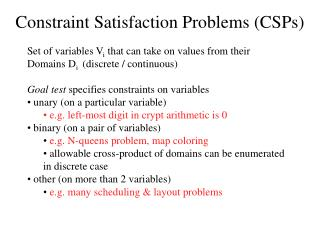# Constraint Satisfaction Problems (CSPs) - PowerPoint PPT PresentationDownload PresentationConstraint Satisfaction Problems (CSPs)

Constraint Satisfaction Problems (CSPs)Download Presentation## Constraint Satisfaction Problems (CSPs)

- - - - - - - - - - - - - - - - - - - - - - - - - - - E N D - - - - - - - - - - - - - - - - - - - - - - - - - - -
##### Presentation Transcript

1. Constraint Satisfaction Problems (CSPs) • Set of variables Vi that can take on values from their Domains Di (discrete / continuous) • Goal test specifies constraints on variables • unary (on a particular variable) • e.g. left-most digit in crypt arithmetic is 0 • binary (on a pair of variables) • e.g. N-queens problem, map coloring • allowable cross-product of domains can be enumerated in discrete case • other (on more than 2 variables) • e.g. many scheduling & layout problems

2. Regularly , but in CSPs, order of assignments does not matter, so we can assign one variable at a time, starting from V1 CSPs … • Depth of search tree = # variables • Can use depth-first search Example: Dshirt = {BLUE, GREEN} Dpants = {RED, YELLOW}

3. Example: Map coloring B A GREEN RED C E F D iDi = { RED, GREEN, BLUE } A map-coloring problem after the first two variables (A and B) have been assigned values Which variable (country) to choose next? If we choose C, which value should we pick?

4. Using problem structure in CSPs Straightforward depth-first-search will not always be efficient • use problem structure to improve (even this seems to not always be effective: CSPs include as subcases known NP-complete problems (e.g. 3SAT)) • Backtracking: Prune the path if constraints violated • Forward checking: Each time a variable is instantiated, delete from the domains of uninstantiated variables all values that conflict with variables assigned so far. Backtrack if some domain becomes empty.E.g. if queens on six columns attack all squares on eighth column. • Arc consistency checking (forward checking is a special case). A state is arc consistent if every variable has a value (or none) that is consistent with the constraints. Successive elimination of values from domains. As preprocessing or during search.

5. Using problem structure in CSPs… • 4. Most-constrained-variable heuristic: • Choose a variable with fewest remaining possible values to instantiate next • - Reduces the branching factor • - Solves “bottleneck” variables early • 5. Most-constraining-variable heuristic: • Choose the variable that participates in the largest number of constraints on un-instantiated variables • - Reduces the branching factor. • Least-constraining-value heuristic: • Choose a value that rules out the smallest number of values in variables connected to the current variable by constraints • CSPs can alternatively be solved using iterative improvement search methods